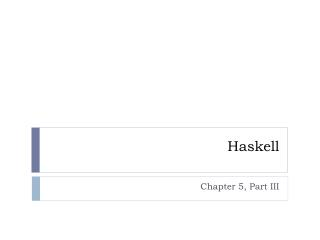Download Presentation# Haskell - PowerPoint PPT Presentation

Haskell. Chapter 5, Part III. Function Application with \$. \$ is the function application operator (\$) :: (a -> b) -> a -> b f \$ x = f x It’s function application but: normal function application has high precedence, \$ has low precedence normal function application is left associate, e.g.,I am the owner, or an agent authorized to act on behalf of the owner, of the copyrighted work described.
Download PresentationAn Image/Link below is provided (as is) to download presentation

Download Policy: Content on the Website is provided to you AS IS for your information and personal use and may not be sold / licensed / shared on other websites without getting consent from its author.While downloading, if for some reason you are not able to download a presentation, the publisher may have deleted the file from their server.

- - - - - - - - - - - - - - - - - - - - - - - - - - E N D - - - - - - - - - - - - - - - - - - - - - - - - - -
Presentation Transcript
1. Haskell Chapter 5, Part III

2. Function Application with \$ • \$ is the function application operator (\$) :: (a -> b) -> a -> b f \$ x = f x • It’s function application but: • normal function application has high precedence, \$ has low precedence • normal function application is left associate, e.g., • f a b c === ((f a) b) c • \$ is right associative • *Main> (^2) 4 + 3 • 19 • *Main> (^2) \$ 4 + 3 • 49

3. Improved syntax with \$ • Most often it’s a convenience that lets us write fewer parentheses. Example: • sum (map sqrt [1..130]) • sum \$ map sqrt [1..130] • when \$ is encountered, expression on right is used as parameter to function on left

4. More examples • sqrt (3+4+9) • sqrt \$ 3+4+9 • *Main> sum (filter (> 10) (map (*2) [2..10])) • 80 • *Main> sum \$ filter (>10) (map (*2) [2..10]) • 80 • *Main> sum \$ filter (>10) \$ map (*2) [2..10] • 80

5. Another example • *Main>(10*) \$ 3 • 30 • *Main> (\$ 3) (10*) • 30 • *Main> map (\$ 3) [(4+), (10*), (^2), sqrt] • [7.0,30.0,9.0,1.7320508075688772] • How does this work? • expression on right is used as parameter to function on left

6. Function Composition – what? • In mathematics, (f o g) (x) = f(g(x)) • Call g with some value, call f with the result (.) :: (b -> c) -> (a -> b) -> a -> c f . g = \x -> f (g x) • parameter of f must be the same as the return type of g • Example: • (negate . (*3)) 4 What’s the answer?

7. Function Composition – Why? • Often convenient to create functions on the fly • Could use lambda, but composition may be more concise • *Main> map (\x -> negate (abs x)) [5,-3, -2, 7] • [-5,-3,-2,-7] • *Main> map (negate . abs) [5, -3, -2, 7] • [-5,-3,-2,-7] • *Main> map (\xs -> negate (sum (tail xs))) [[1..5],[3..6],[1..7]] • [-14,-15,-27] • *Main> map (negate . sum . tail) [[1..5],[3..6],[1..7]] • [-14,-15,-27] Who can explain this answer?

8. Function composition with multiple parameters • If a function takes multiple parameters, must partially apply • *Main> sum (replicate 5 (max 6 9)) • 45 • *Main> (sum . replicate 5) (max 6 9) • 45 • *Main> sum . replicate 5 \$ max 6 9 • 45 How does this syntax capture semantics?

9. The process • To rewrite a function with lots of parentheses using function composition • first write out the innermost function and its parameters • then put a \$ before it • compose all prior functions by omitting their last parameter (but not other parameters) and putting . between them • *Main> replicate2 (product (map(*3) (zipWith max [1,2] [4,5]))) • [180,180] • *Main> replicate 2 . product . map (*3) \$ zipWith max [1,2] [4,5] • [180,180]

10. Play and Share Using \$ • write bigCubes that takes a list and returns a list of cubes that are > 500 • write lottaBiggest that takes a list and replicates the largest element 4 times. lottaBiggest [2,5,3,1] => [5,5,5,5] • write powers that takes a number and creates a list of that number squared, cubed, and quadrupled. powers 2 => [4,8,16] • Assume people are dining. We have a list of tip percents (assume people tip at different rates): • *Main> let pcts = [0.15, 0.2, 0.21] • We have a list of bills (what people owe, minus tip) • *Main> let amts = [20.5, 30, 25] • Write calcBill that takes amts and pcts and calculates what each person will pay, based on their amt and pct. Then apply a 4% tax rate. • *Main>calcBillamtspcts • [24.518,37.44,31.46] • Hint: I did this first with (), then converted to remove (). I used zipWith twice (your soln may vary). (tax added)

11. Play and Share continued Using . and/or \$ • write overtimePay that takes a rate a list of hours and calculates the amount of overtime pay. Same hint as above. Ex: overtimePay 20 [50,30,40] => 200 • write splitBill that takes an amount, adds 25% to cover tax and tip, and splits the bill between two diners. splitBill 50 => 31.25